## 3.6 Modulation synthesis

### 3.6.1 Theory

#### 3.6.1.1 Ring modulation

Let's first look at this phenomenon: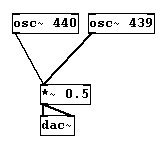If you listen to two sine tones very close to each other, you hear fluctuating wave cancellations. This is due to the interaction of two almost (but only almost!) identical waves. This phenomenon is called beating. The speed, or rhythm, of the beating is exactly equal to the difference between the two frequencies - in this example: 440 - 439 = 1 Hz.

Let's take a look at a simplified example using oscillators with 4 and 5 Hz: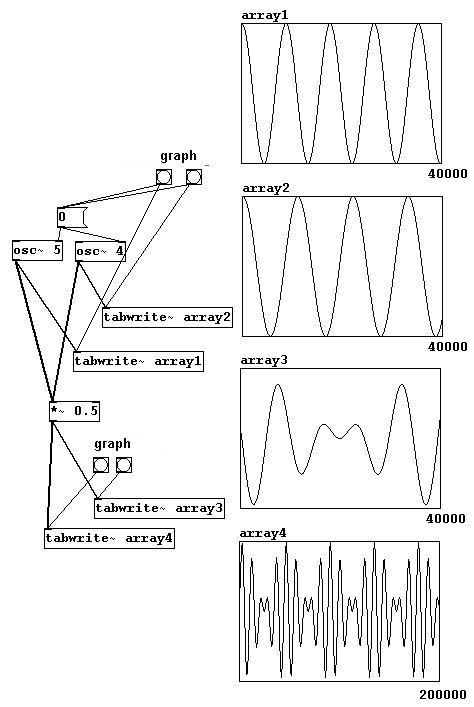The waves alternate between summations (never forget: waves add themselves together!) and cancellations. In array1 and array2 you can see a part of the original wave, in array3 the summation, and in array4 the summation again over a longer period of time. The result is a pulsing rise and fall in volume. (For the human ear to perceive two different tones, their frequencies differ by about five cents.)

You can also first determine, precisely, the rhythm of these amplitude fluctuations. As you saw in array4 in the previous diagram, the amplitude has a sinusoid shape. You could therefore simply use an oscillator to determine the amplitude: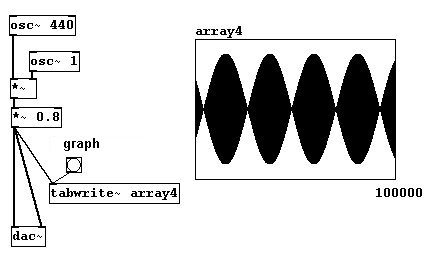(The reason this array is so dark in comparison to the previous one is because it uses much higher frequencies.) The resulting wave corresponds to the summation of two waves. If you raise the frequency of the modulating amplitude higher and higher......you hear two frequencies that move away from each other symmetrically - one up, one down - by an interval equal to the distance of the amplitude from the middle axis, i.e., the initial amplitude frequency. This process is an example of amplitude modulation, called 'ring modulation' because of its symmetrical nature. If the initial frequency is 440 Hz and the amplitude frequency is 100 Hz, you'll hear two tones: one at 340 Hz and one at 540 Hz.

#### 3.6.1.2 Frequency modulation

You could also use an oscillator to modulate a sine wave's frequency. This is called frequency modulation: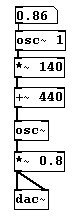One oscillator is "carrier" and the other is "modulator". Using a low frequency with the "modulator" will result in vibrato. Beginning at 20 Hz, increasing this frequency will result in a more and more complex multiphonic: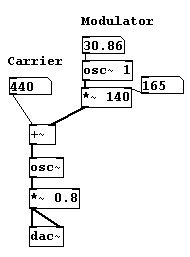The resulting wave is the summation of many different sine waves; the carrier frequency lies in the middle while the other tones lie above and below it at distances determined by the modulation frequency.When the modulation amplitude rises, the amplitudes of the additional frequencies also rise. However, this increase is difficult to formulate mathematically.

A special situation arises when the modulation frequency is a whole number multiple of the carrier frequency (i.e., 1x, 2x, 3x, 4x, 5x, 6x, etc.). The other tones above the carrier frequency would also be whole number multiples of the carrier frequency - i.e., its overtones.

Furthermore negative frequencies are mirrored above in the positive range. In the special situation mentioned in the previous paragraph, these are covered by "normal" frequencies. Let's say you have a carrier frequency of 200 Hz and a modulator frequency of 100 Hz; coverings occur starting with the third undertone (which is also 100 Hz and the following 200, 300, etc.), which result in amplifications and suppressions according to phase length.

The advantage of FM synthesis over additive synthesis (the simple addition of sine waves) is that you only need two oscillators to make a rich and complex sound (you just have to change the frequency and especially the amplitude of the modulator!). A typical FM synthesis sound is a 'disharmonic spectrum', i.e., a quasi-spectrum above the fundamental with distorted overtones that are not whole number multiples of the fundamental. Some metallic instruments, like bells and gongs, exhibit similar spectra; sounds made using FM synthesis often have a 'metallic' timbre for this reason.

### 3.6.2 Applications

#### 3.6.2.1 More sonically complex ring modulation

Ring modulations used with overtone-rich sounds are naturally much more complex and rich: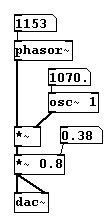#### 3.6.2.2 Live ring modulation#### 3.6.2.3 Live frequency modulation

To use frequency modulation in live settings, you have to use variable delay in order to be able to change the frequency: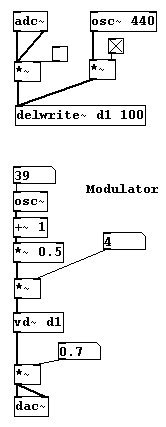#### 3.6.2.4 More exercises

Combine everything you've learned up to now.

### 3.6.3 Appendix

#### 3.6.3.1 Phase modulation

Frequency modulation is also called phase modulation and can also be programmed in this form. To accomplish this, the carrier oscillator must be divided into phase processing and waveform processing. Here's how that works:And the phase modulation looks like this: